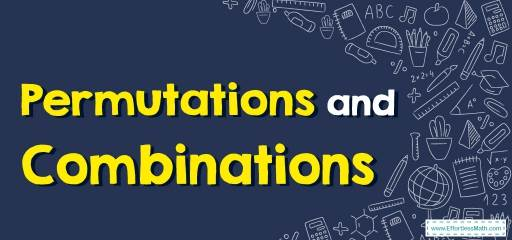# How to Solve Permutations and Combinations? (+FREE Worksheet!)

Learn how to solve mathematics word problems containing Permutations and Combinations using formulas.## Step by step guide to solve Permutations and Combinations

• Permutations: The number of ways to choose a sample of $$k$$ elements from a set of $$n$$ distinct objects where order does matter, and replacements are not allowed. For a permutation problem, use this formula:
$$\color{blue}{_{n}P_{k }= \frac{n!}{(n-k)!}}$$
• Combination: The number of ways to choose a sample of $$r$$ elements from a set of $$n$$ distinct objects where order does not matter, and replacements are not allowed. For a combination problem, use this formula:
$$\color{blue}{_{ n}C_{r }= \frac{n!}{r! (n-r)!}}$$
• Factorials are products, indicated by an exclamation mark. For example, $$4!$$ Equals: $$4×3×2×1$$. Remember that $$0!$$ is defined to be equal to $$1$$.

A Perfect Practice Workbook for Algebra I

### Permutations and Combinations – Example 1:

How many ways can the first and second place be awarded to $$10$$ people?

Solution:

Since the order matters, we need to use the permutation formula where $$n$$ is $$10$$ and $$k$$ is $$2$$. Then: $$\frac{n!}{(n-k)!}=\frac{10!}{(10-2)!}=\frac{10!}{8!}=\frac{10×9×8!}{8!}$$, remove $$8!$$ from both sides of the fraction. Then: $$\frac{10×9×8!}{8!}=10×9=90$$

### Permutations and Combinations – Example 2:

How many ways can we pick a team of $$3$$ people from a group of $$8$$?

Solution:

Since the order doesn’t matter, we need to use a combination formula where $$n$$ is $$8$$ and $$r$$ is $$3$$. Then: $$\frac{n!}{r! (n-r)!}=\frac{8!}{3! (8-3)!}=\frac{8!}{3! (5)!}=\frac{8×7×6×5!}{3! (5)!}$$, remove $$5!$$ from both sides of the fraction. Then: $$\frac{8×7×6}{3×2×1}=\frac{336}{6}=56$$

## Exercises for Solving Permutations and Combinations

Best Algebra Prep Resource

### Calculate the value of each.

1. $$\color{blue}{4!=}$$
2. $$\color{blue}{4!×3!=}$$
3. $$\color{blue}{5!=}$$
4. $$\color{blue}{6!+3!=}$$
5. There are $$7$$ horses in a race. In how many different orders can the horses finish?
6. In how many ways can $$6$$ people be arranged in a row?

1. $$\color{blue}{24}$$
2. $$\color{blue}{144}$$
3. $$\color{blue}{120}$$
4. $$\color{blue}{726}$$
5. $$\color{blue}{5,040}$$
6. $$\color{blue}{720}$$

The Absolute Best Books to Ace Algebra

### What people say about "How to Solve Permutations and Combinations? (+FREE Worksheet!) - Effortless Math: We Help Students Learn to LOVE Mathematics"?

No one replied yet.

X
45% OFF

Limited time only!

Save Over 45%

SAVE $40 It was$89.99 now it is \$49.99